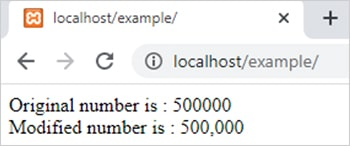number_format() function in PHP || PHP number_format() function# number_format() function in php

1746

PHP number_fomat() function is used to format a number with grouped thousands. It is an inbuilt function of PHP. The return type of this function is number means it returns a formatted number corresponding to the given number.

Syntax:

number_format(\$number,\$decimal,\$decimal_point,\$separator_char);
• \$number is a number that is formatted by this function. It is mandatory.
• \$decimal is used to decide the position of the decimal point. It is optional.
• \$decimal_point represents a string which used for the decimal point. It is also optional.
• \$separater_char represents which character is used to separate thousands. If it is a string then only the first character of string used as a separator. It is also optional but if this parameter is given then all the above parameters are required to be given.

Example:

```<?php
\$number=500000;
\$modified_number=number_format(\$number);
echo "Original number is : \$number <br>";
echo "Modified number is : \$modified_number";
?>```

Output:Example 2:

```<?php
\$number=730550;
\$modified_number=number_format(\$number,2); // rounded with 2 decimal position
echo "Original number is : \$number <br>";
echo "Modified number is : \$modified_number";
?>```

Output:Share:## Big Hosting Days

Upto 75@ OFF on Web Hosting

## Get a .COM for just \$6.98

Secure Domain for a Mini Price